# Determining the Pressure and Altitude Using GY-68 BMP180 and GPL3+

### Содержание

In this tutorial, you’ll learn how to use the BMP180 sensor, which is one of the most commonly used sensors for measuring the pressure.

• 11,168 views
• 6 respects

## Apps and online services

In many projects such as flying robots, weather stations, improving routing performance, sports and etc. measuring pressure and altitude is very important. In this tutorial, you’ll learn how to use the BMP180 sensor, which is one of the most commonly used sensors for measuring the pressure.

What You Will Learn

• What the barometric pressure is.
• What the BOSCH BMP180 pressure sensor is.
• How to use the BOSCH BMP180 pressure sensor with Arduino.

What Is the Barometric Pressure?

Barometric pressure or atmospheric pressure results from the weight of the air on the earth. This pressure is about 1 kg per square centimeter at the sea level.

There are several units to express the atmospheric pressure, that can easily be converted to each other. The SI unit for measuring the pressure is Pascal (Pa).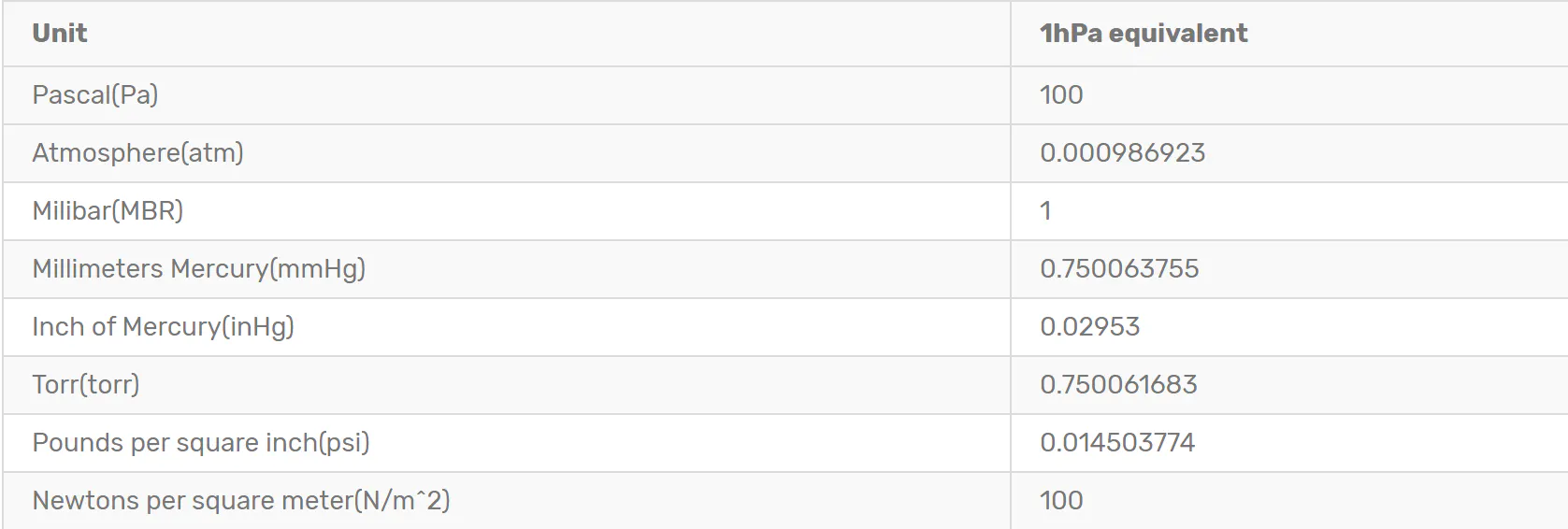The barometric pressure has an approximately linear inverse ratio with the altitude from the sea level so if we measure the barometric pressure of a place, we can calculate the altitude from the sea level using a simple mathematical operation.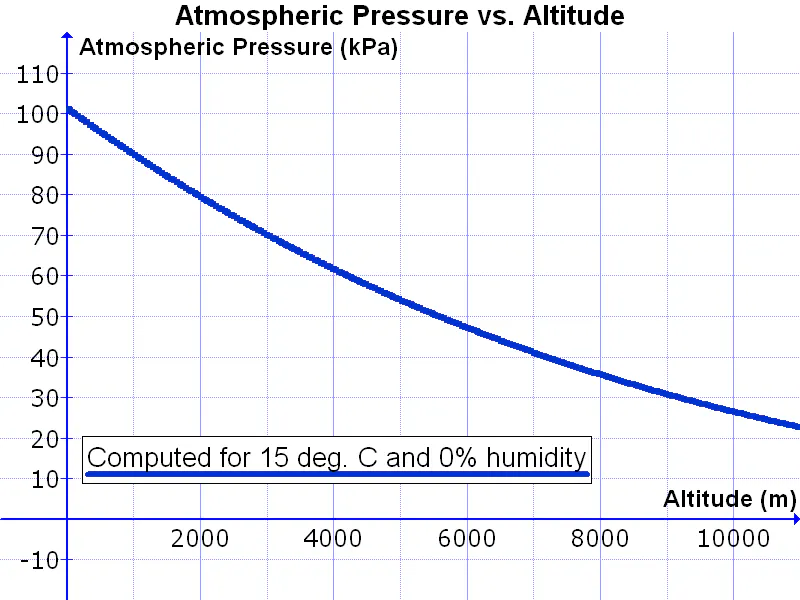GY-68 BOSCH BMP180 Pressure Sensor Features

One of the most common sensors for measuring the pressure and altitude is BOSCH BMP180. The most important features of this module are as follow:

• Pressure measurement range of 300 to 1100hPa
• -0.1hPa measuring accuracy for absolute pressure
• 12hPa measuring accuracy for relative pressure
• Low power consumption (5μA in standard mode and one sample per second)
• Internal temperature sensor with a precision of 0.5 °C
• Supporting I2C protocol for communication
• Fully calibrated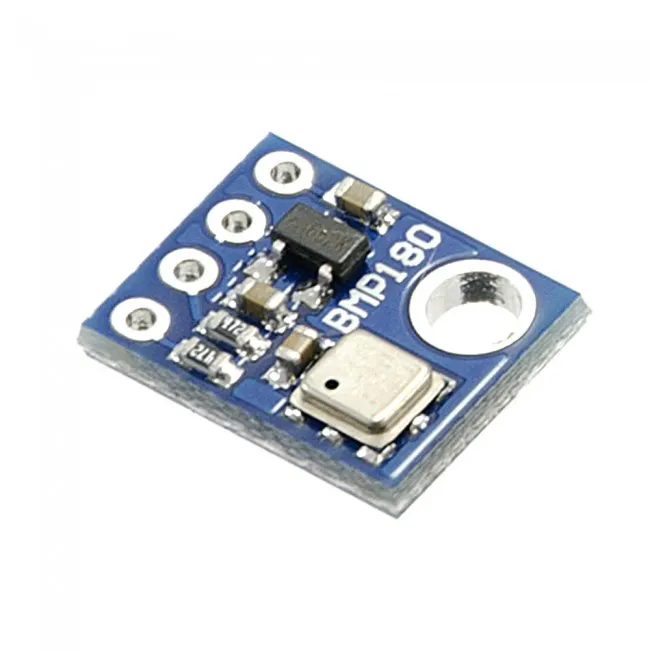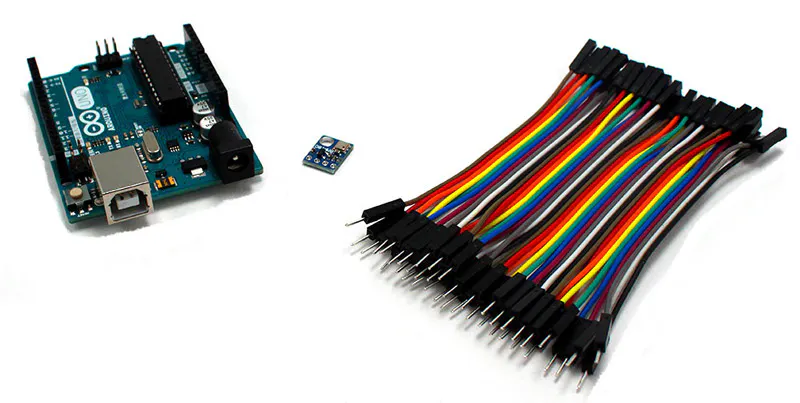How to Use GY-68 BMP180 Pressure Sensor with Arduino?

This sensor is available as a module for easy use. The main parts of the BMP180 sensor module are: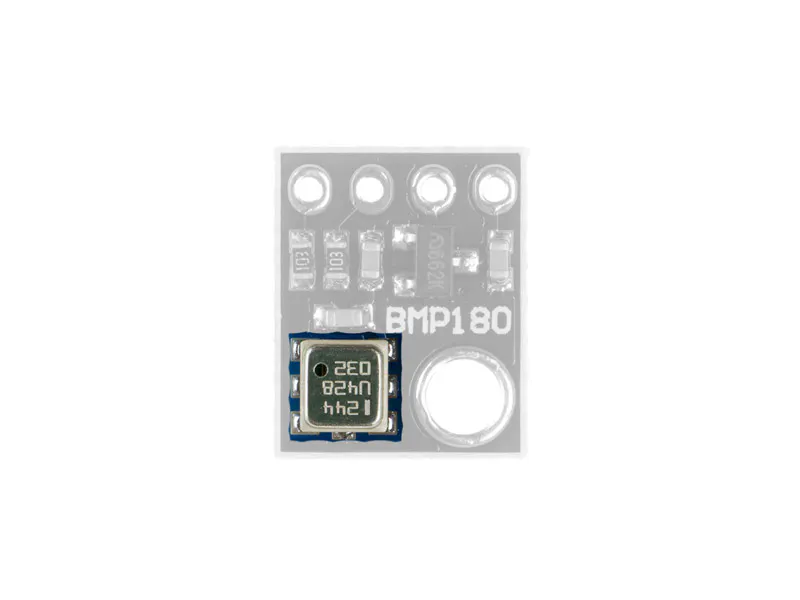• A 3.3-volt regulator. This regulator lets you connect the module to 5V.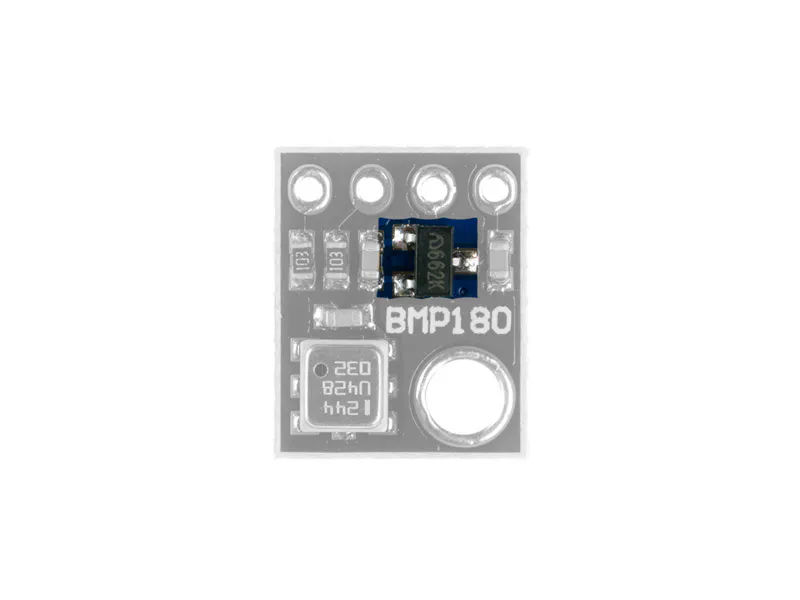• Required pull up resistors to communicate I2C properly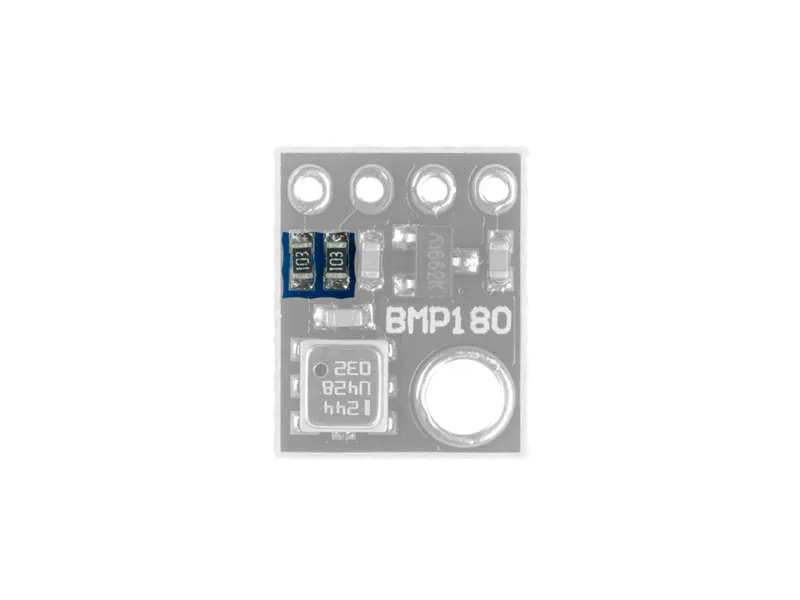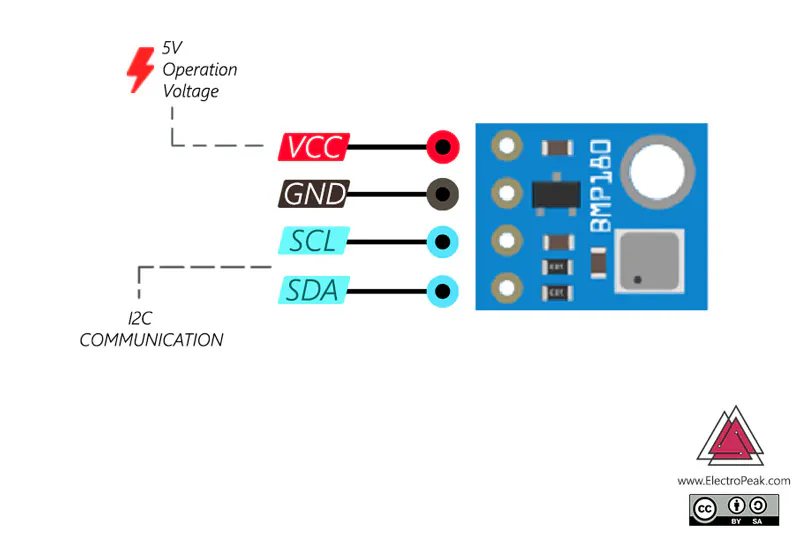Download the BMP180_Breakout_Arduino_Library to use the BMP180 sensor module.

Calculation of Absolute Pressure with Different Units and Altitude from the Sea Level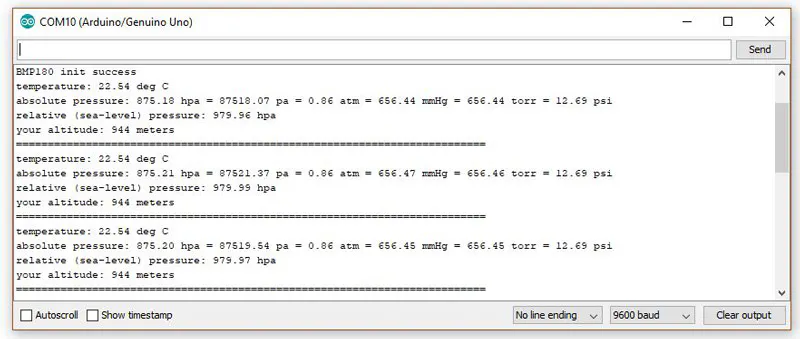Let’s check the process of pressure and altitude calculation more accurately: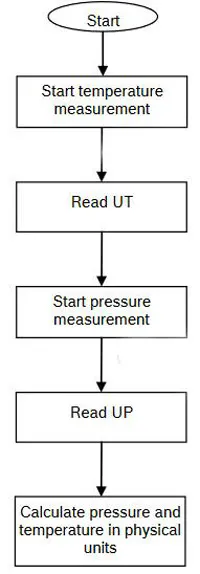According to the above algorithm, first we start to calculate the temperature using startTemperature() , then we store the temperature in variable T using getTemperature(T) . After that, we calculate the pressure with startPressure(3) . The number 3 is the maximum resolution that can be changed between 0 and 3. using getPressure(P) we store the absolute pressure in variable P. The amount of this pressure is in hPa, which can be converted to different units according to the previous table. The absolute pressure changes with altitude. To remove the effect of the altitude on the calculated pressure, we should use the sealevel(P, ALTITUDE) function according to the altitude stored in the ALTITUDE variable, and store the measured value in an arbitrary variable, such as p0. Use altitude(P, p0) to calculate your altitude. This function calculates the altitude in the meter.

Note that you can insert your altitude from the sea level for the ALTITUDE variable defined at the beginning of the code

Make an Altimeter!

The BMP180 pressure sensor, like many other pressure sensors, measures the absolute pressure, and the absolute pressure changes with the change of the altitude. So you can calculate the relative altitude by measuring the pressure.

The startPressure(3) function calculates the absolute pressure value. Since the pressure changes with the change of the temperature and humidity, we store the initial pressure in baseline variable. According to this value and instantaneous pressure, the altitude(P, baseline) function calculates the relative altitude in meter.

Источник: create.arduino.cc